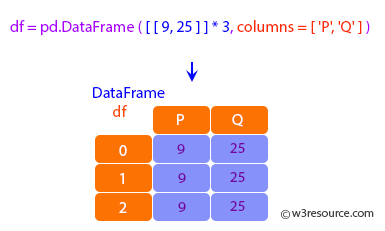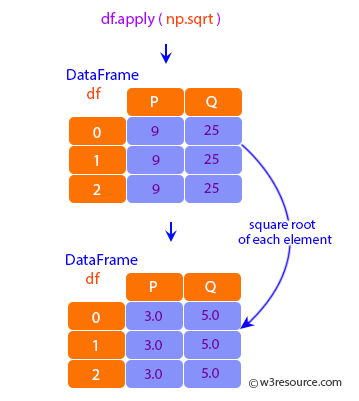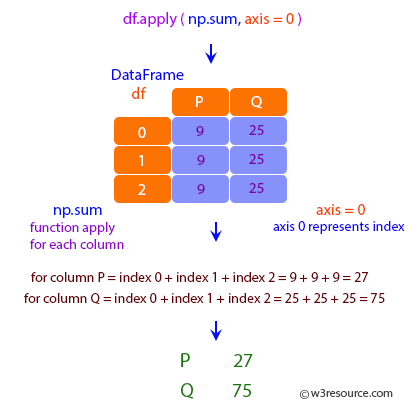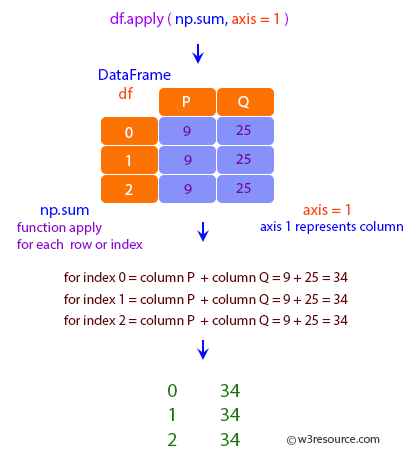Examples

In :
import numpy as np
import pandas as pd

In :
df = pd.DataFrame([[9, 25]] * 3, columns=['P', 'Q'])
df

Out:
P Q
0 9 25
1 9 25
2 9 25Using a numpy universal function (in this case the same as np.sqrt(df)):

In :
df.apply(np.sqrt)

Out:
P Q
0 3.0 5.0
1 3.0 5.0
2 3.0 5.0Using a reducing function on either axis

In :
df.apply(np.sum, axis=0)

Out:
P    27
Q    75
dtype: int64In :
df.apply(np.sum, axis=1)

Out:
0    34
1    34
2    34
dtype: int64Returning a list-like will result in a Series:

In :
df.apply(lambda x: [1, 2], axis=1)

Out:
0    [1, 2]
1    [1, 2]
2    [1, 2]
dtype: object

Passing result_type=’expand’ will expand list-like results to columns of a Dataframe:

In :
df.apply(lambda x: [1, 2], axis=1, result_type='expand')

Out:
0 1
0 1 2
1 1 2
2 1 2

Returning a Series inside the function is similar to passing result_type='expand'.
The resulting column names will be the Series index.

In :
df.apply(lambda x: pd.Series([1, 2], index=['f1', 'b1']), axis=1)

Out:
f1 b1
0 1 2
1 1 2
2 1 2

Passing result_type='broadcast' will ensure the same shape result, whether list-like
or scalar is returned by the function, and broadcast it along the axis. The resulting
column names will be the originals.

In :
df.apply(lambda x: [1, 2], axis=1, result_type='broadcast')

Out:
P Q
0 1 2
1 1 2
2 1 2